Mathematical and Physical Journal
for High Schools
Issued by the MATFUND Foundation
 Already signed up? New to KöMaL?

# KöMaL Problems in Physics, October 2008

Show/hide problems of signs:## Problems with sign 'M'

Deadline expired on November 11, 2008.

M. 290. Measure the magnitude of the torque needed to screw down the top of a plastic water or soft drink bottle. How does this depend on the maximum torque applied during the unscrewing process of the top?

(6 pont)

statistics## Problems with sign 'P'

Deadline expired on November 11, 2008.

P. 4094. The hinged rod of weight G shown in the figure is supported by the horizontal, slippery floor. Find the magnitude and the direction of the forces acting at the hinge and at the floor.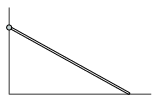(3 pont)

statistics

P. 4095. The traffic in some part of a three-lane motorway is restricted to only one lane. In this part the cars move uniformly at a speed of 54 km/h and 11-m long gaps are formed between them.

a) What is the speed of the cars before the one-lane part, if the gap between them is 2 m?

b) What will the length of the gaps between the cars be after the one-lane part if the cars speed up to a velocity of 126 km/h?

Let us consider the length of each car 4 m.

(4 pont)

statistics

P. 4096. An object is 20 cm away from a concave mirror, and it is within the focal length of the mirror. If the mirror is changed to a plane mirror the image moves 15 cm closer to the mirror.

a) What is the focal length of the concave mirror?

b) Calculate the enlargement.

(3 pont)

statistics

P. 4097. The wedge, shown in the figure, has a mass of m and an angle ofand can slide without friction along the horizontal tabletop. A block of mass m is placed onto the wedge, and it slides down without friction. At what anglewill the magnitude of the acceleration of the wedge be at least one-third of the acceleration due to gravity?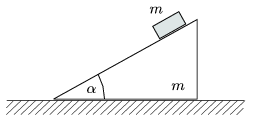(5 pont)

statistics

P. 4098. A spaceship gets into an interplanetary dust cloud. The average mass of the grains of the cloud in a cubic metre is is 0.02 mg. The speed of the spaceship with respect to the cloud is 10 km/s, the area of its cross section which is perpendicular to its speed is 50 m2. The collision between the grains and the spaceship is totally inelastic. What must the thrust of the engine be in order not to change the speed of the spaceship?

(4 pont)

statistics

P. 4099. A sample of water of mass m1 and of temperature t1 is in a thermos flask, and warmer water of mass m2 and of temperature t2 is poured to it. After the themal equilibrium is reached the temperature is measured. Next time, because we are curious, we swop the two samples of water, so the colder water of mass m1 and of temperature t1 is poured into the thermos in which there is the other sample of water of mass m2 and of temperature t2. Surprisingly it is found that the equilibrium temperature in the second case is different from the one measured in the first time.

a) How can you explain this difference?

b) The difference between the two equilibrium temperatures is 1.9 oC. What can you deduce from this?

Data: m1=300 g, t1=20 oC, m2=600 g, t2=80 oC.

(4 pont)

statistics

P. 4100. A wall divides the cylindrical, thermally insulated container of volume 20 litres into two parts as shown in the figure. There is vacuum in the left part which is closed by a thermally insulated fixed piston, and in the other part there is Nitrogen gas at a pressure of p=5.105 and at a temperature of 300 K. (The external pressure is 105 Pa.) The door on the wall opens because of the excess pressure, and the gas expands immediately. Then the fixing of the piston is ceased, and the gas is pushed back into its original place.

a) How much work was performed during the compression of the gas?

b) What are the temperature and the pressure of the gas at the end of the process?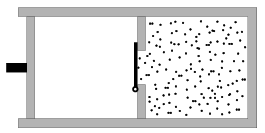(5 pont)

statistics

P. 4101. Several batteries, all having the same electromotive forces and the same internal resistances, were connected first in parallel and then in series and a resistor of resistance 129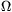was connected into the circuit. It was found that in case of the series connection the terminal voltage across each battery is 10% less than in case of the parallel connection. How many batteries were used?

(4 pont)

statistics

P. 4102. Is it possible that an electron undergoes uniform straight line motion while it travels through a region of perpendicularly crossed electric and magnetic fields? The electric field is E=3 kV/m and the magnetic induction is B=10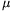T.

(4 pont)

statistics

P. 4103. The mass of a two-engine plane is m. Because of some breakdown there is a considerable difference between the thrusts of the engines, which is. Despite of the breakdown the pilot managed to drive the plane horizontally along a straight line.

a) What is the force exerted on the vertical surface of the tail if the distance between the tail and the centre of mass is one and a half times the distance between the two engines?

b) Why and by what angle did the pilot have to lean the plane?

The plane is symmetrical, and it can be assumed that after the breakdown air resistance is exerted at the plane of symmetry.

(5 pont)

statistics